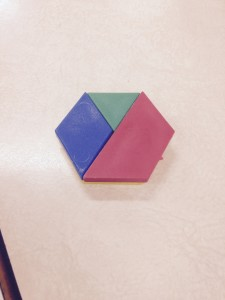# Understanding Equality Using Pattern BlocksRecently a primary teacher asked me for suggestions for hands-on activities for balance and equality.  As I mentioned in a previous post we have a tendency to focus more on patterning and less on algebra, but here is a Grade 1 teacher understanding the importance of moving beyond.  In response to her request I told her that I like to build understanding of equality using concrete materials before moving on to numbers.  To do this I have often used the following task with students from K-6:

How many different ways can you make the yellow hexagon using other pattern blocks?

There are several different ways, therefore, I bring out the idea of equality through the student work. The combinations  equivalent to the yellow hexagon are:

• 2 red trapezoids
• 3 blue rhombuses
• 6 green triangles
• 1 red trapezoid, 1 blue rhombus , 1 green triangle
• 1 red trapezoid, 3 green triangles
• 1 blue rhombus, 4 green triangles
• 2 blue rhombuses, 2 green triangles

By spending time creating these different hexagons the students are exploring the relationships between the pattern blocks which will ultimately impact their understanding of fractions and proportional reasoning.  For example, if it takes 2 red trapezoids to make the yellow hexagon, then the trapezoid is one-half the size of the hexagon or the hexagon is twice as big as the trapezoid.  Soon some students will be ready to work with numbers so I might ask:  If the hexagon is worth 6, what would the other pattern blocks be worth? (triangle-1, rhombus-2, trapezoid-3). If the hexagon is now worth 12, how does that affect the value of the other pattern blocks?Pattern blocks help us visualize what 1/2+1/3+1/6 is equal to.

What is 1/2+1/3+1/6 = ?

Do you have to find common denominators to figure this out?